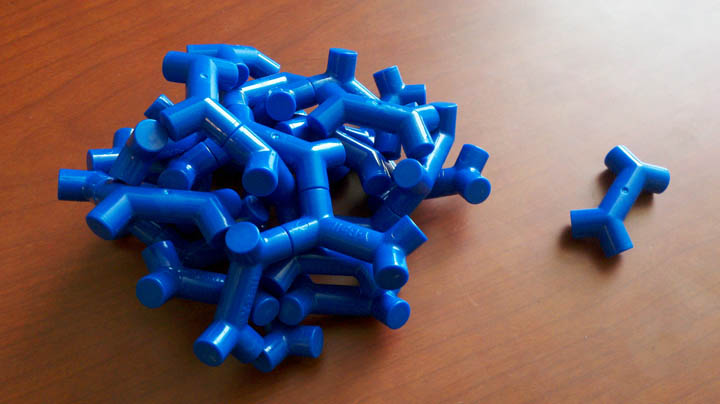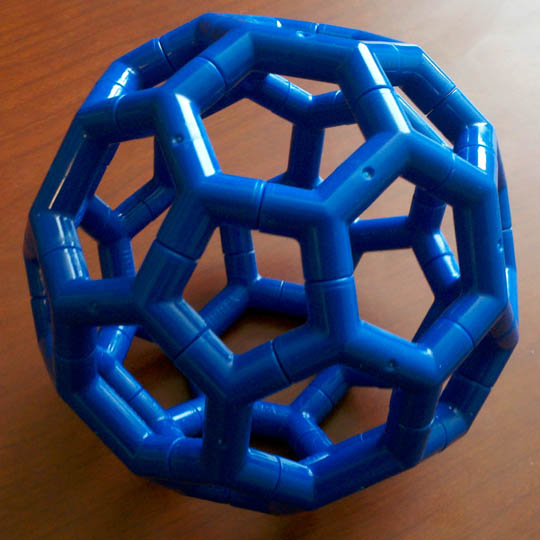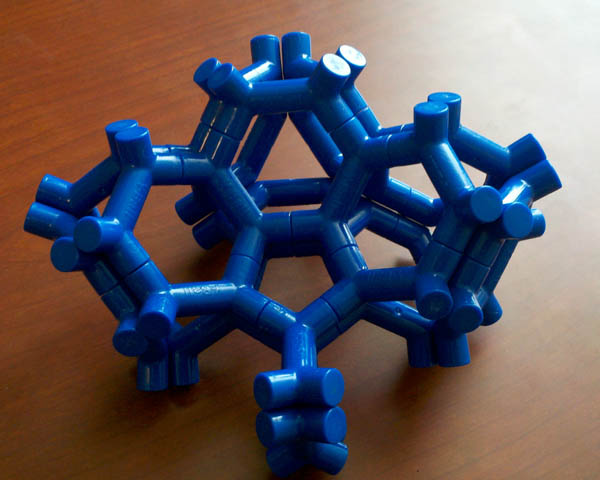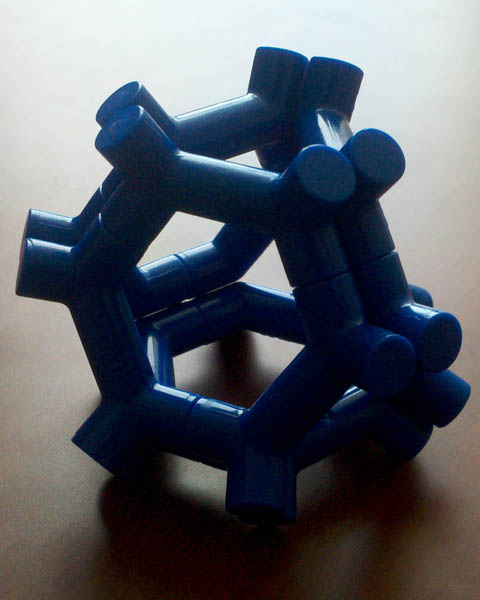really like to connect up to each other in the form of a buckyball (a truncated icosahedron):But that's far from the only pattern they can form. Here's part of another:I only have enough of the little pieces to form one buckyball, but it looks as if this pattern could be continued indefinitely, forming a collection of multiple buckyballs with their pentagonal faces magnetically attached to each other. The gaps between the buckyballs look like truncated tetrahedra; here's a single gap all by itself:Unfortunately, appearances can be deceiving. The angles between the buckyball faces don't quite add up to match the angles between the faces of the truncated tetrahedra, so the sum of the angles surrounding each edge is not 360 degrees, as it should be, but rather 357 or 355 degrees (depending on which of the two kinds of edges it is: one formed by two magnets, or by four). So if you tried to extend this pattern far enough in Euclidean space, you'd run into serious problems with its geometry.

Fortunately, we don't have only Euclidean space to work with: we can also use other geometries, such as hyperbolic or spherical space. In hyperbolic geometry, the dihedral angles of a polyhedron such as a truncated icosahedron are sharper than they would be in Euclidean space; in spherical geometry it's the opposite. For this pattern, because its angle sums are too small (rather than too large), we should use a spherical geometry. And it turns out that in spherical geometry, unlike Euclidean geometry, there is a way to fill space with truncated icosahedra and truncated tetrahedra. It's a four-dimensional convex polytope, known as the bitruncated 120-cell, and it has 120 truncated icosahedron faces and 600 truncated-tetrahedron faces, meeting in exactly the pattern shown to us by these magnets. So if only our universe were a 3-sphere of small enough size (or if the magnetic pieces were of cosmological scale) we could build this structure in such a way that we could see the whole thing surrounding us, partitioning space into 720 rooms of two different shapes.